# Beurling-Lax theorem

(diff) ← Older revision | Latest revision (diff) | Newer revision → (diff)

A theorem involved with the characterization of shift-invariant subspaces of the Hardy spaceof analytic functions on the unit disc in terms of inner functions (cf. also Hardy spaces). Specifically, the space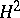can be characterized as the space of analytic functionson the unit disc having Taylor series representationwith square-summable coefficients (i.e.,). The shift operatoronis defined to be the operator of multiplication by the coordinate function: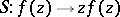. A closed linear subspace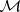ofis said to be invariant forif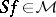whenever. In 1949 (see [a6]), A. Beurling proved that any such shift-invariant subspace has the formwhereis an inner function. Here, an inner function is an analytic function on the unit disc with contractive values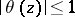such that its boundary values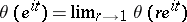(which exist for almost every pointwith respect to Lebesgue measure on the unit circle) have modulus(i.e.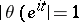) for almost all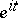. The usefulness of the result is enhanced by the fact that such inner functions can be factored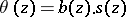, where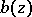is a Blaschke product which collects all the zeros of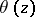in the unit disc, and a singular inner function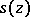, given by an explicit integral formula in terms of a singular measure on the unit circle. When one applies the result to the cyclic invariant subspacegenerated by a given functionin, one arrives at the inner-outer factorization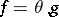of. Here, the right factor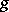is an outer function; such functions are characterized operator-theoretically as the cyclic vectors for the operator, or function-theoretically by an integral representation formula arising from an absolutely continuous measure on the unit circle. P.D. Lax [a11] extended the result to finite-dimensional vector-valued(in the alternative setting whereof the unit disc is replaced byof the right half-plane). Later, P.R. Halmos [a12] gave an elegant proof for the infinite-dimensional case. V.P. Potapov [a15] worked out an analogue of the parametrization (in terms of zeros and a singular measure on the circle) of an inner function for the matrix-valued case. It turns out that the parametrization of matrix inner functions (and, more generally, of contractive analytic matrix-functions on the unit disc) in terms of Schur parameters (see [a9] and [a16]) has proved more useful for engineering applications.

Generalizations and applications of this result have been going strong (with some interruptions) to the present day (1998). Beurling already observed that the detailed parametrization of inner functions (in terms of zero locations and singular measures on the unit circle as sketched above) leads to a complete characterization of the lattice structure of the lattice of invariant subspaces for the shift operator(a strikingly different structure from the previously worked out case of self-adjoint or unitary operators). In the 1960s, operator theorists realized that the compressionof the shift operatorto the orthogonal complementof a shift-invariant subspace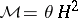serves as a model for a rich class of Hilbert-space operators. The model theories of L. de Branges and J. Rovnyak [a7] and that of B. Sz.-Nagy and C. Foiaş [a18] give two roughly equivalent generalizations of, each of which leads to a model for an arbitrary, completely non-unitary contraction operator on a Hilbert space. In both of these theories, the function(a contractive, operator-valued, analytic function on the unit disc) is called the characteristic function of the associated operator. In the case whereis inner (i.e., the boundary values on the unit circle are isometries almost everywhere), the model space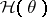reduces to the Beurling–Lax form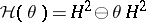. There also has evolved a theory of so-called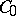operators, for which the operator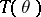(witha scalar inner function) are the building blocks in an analogue of a canonical Jordan form which classifiesoperators up to quasi-similarity (see [a18] and [a5]).

The model theoretical approach of M.S. Livsic and coworkers in the former Soviet Union (see [a8]) gives an alternative formulation with emphasis on the system-theoretic aspects (where the characteristic function appears as the transfer function of a discrete-time, linear, energy-conserving system) rather than on connections with Beurling–Lax representations for shift-invariant subspaces. In 1983, J.A. Ball and J.W. Helton [a3] showed how a new type of Beurling–Lax representation for the vector-valued case (where one demands thatand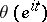preserve an indefinite rather than Hilbert-space inner product) leads directly to the linear-fractional parametrization for the solution set of a Nevanlinna–Pick interpolation problem. Connections with engineering applications, such as signal processing, robust control and system identification, have led to new questions, different points of view, and an emphasis on robust computational procedures; in particular, the model spacecan be interpreted as the range of a controllability operator, and there are explicit procedures for realizingas the transfer functionof a unitary system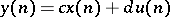from the common zeros of the invariant subspace(see, e.g., [a2] and [a16]). There have been recent extensions of a number of these ideas to time-varying systems (see, e.g., [a10]) and even to non-linear systems (see [a4]).

There have been other extensions from the point of view of function-theoretic operator theory. Already in the 1960s, other workers (such as D. Lowdenslager, H. Helson and J. Wermer) obtained Beurling-type representations for shift-invariant subspaces inor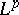, as well as extensions to more abstract harmonic analysis and function-algebra settings. A more delicate Beurling–Lax representation theorem has been shown to hold for Hardy spaces on finitely-connected planar domains, where unitary representations of the fundamental group of the underlying domain play a fundamental role in the description (see, e.g., [a1]). Recently (1990s) there have been attempts to generalize Halmos' wandering subspace construction in still other directions. For example, there is a Beurling-like representation theorem for invariant subspaces of the Dirichlet shift (see [a17]) and a notion of inner divisor for the Bergman space [a14] (cf. also Bergman spaces).

How to Cite This Entry:
Beurling-Lax theorem. Encyclopedia of Mathematics. URL: http://encyclopediaofmath.org/index.php?title=Beurling-Lax_theorem&oldid=17989
This article was adapted from an original article by Joseph A. Ball (originator), which appeared in Encyclopedia of Mathematics - ISBN 1402006098. See original article